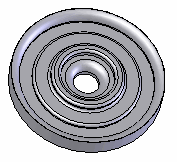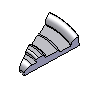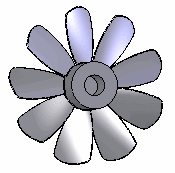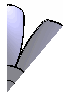Analyzing Circular Models

In many cases, you can take advantage of symmetry conditions to analyze a portion of a circular model instead of the whole model. Here are some guidelines:

• When determining symmetry, consider geometry, materials, restraints, and loads.
• For frequency and buckling studies, analyze the full model. If you analyze a part of the model using symmetry, you get symmetrical modes only.
• For simple models, analyze the full model. The results should be symmetrical.
• If the model is fully axi-symmetric and the loads are such that the cut sections cannot deform normal to their planes, you can analyze a wedge. Use an angle for the wedge that is divisible by 360o. Practically, you should not use a very small angle that can result in a bad mesh especially when there is no hole at the center of the model. Note that you cannot use this option if tangential loads exist since the cut planes can deform normal to their planes. Use cyclic symmetry in such cases.
• If the model has a circular pattern and the loads are such that the cut sections cannot deform normal to their planes, you can analyze half or one quarter of the model to apply Symmetry constraints on the cut section.
• If the loads are such that the cut sections can deform normal to their planes, use Cyclic symmetry.
Cyclic symmetry is more general as it can solve problems where the cut sections can deform in the tangential direction.

For example, you can analyze a wedge of the disc shown below that forms an angle divisible by 360o. In other words, the number of segments that are repeated about the axis of revolution of the symmetry should be an integer number.

If all forces are radial, you can use the Symmetry condition on a half or one quarter cut section.

If tangential loads exist, the cut sections can deform out of their planes and you must use Cyclic symmetry or analyze the full model.For the fan shown below, you can analyze one blade (one ninth of the model). Since loads on the blades are usually tangential, using Cyclic symmetry is recommended.Although any one ninth of the model is a valid pattern, it is recommended to use a pattern that does not cut through the blades.